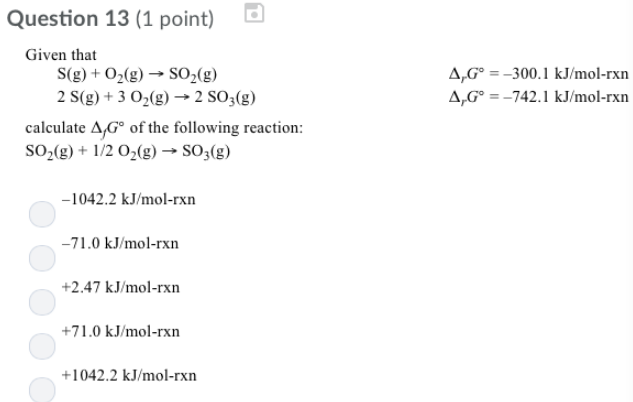# Problem: Given that S (g) + O2 (g) → SO2 (g)           ΔrG° = -300.1 kJ/mol-rxn 2S (g) + 3O2 (g) → 2SO3 (g)    ΔrG° = -742.1 kJ/mol-rxn calculate ΔJG° of the following reaction: SO2 (g) + 1/2O2 (g) → SO3 (g) (a) -1042.2 kJ/mol-rxn (b) -71.0 kJ/mol-rxn (c) +2.47 kJ/mol-rxn (d) +71.0 kJ/mol-rxn (e) +1042.2 kJ/mol-rxn

###### FREE Expert Solution
81% (423 ratings)###### Problem Details

Given that

S (g) + O2 (g) → SO2 (g)           ΔrG° = -300.1 kJ/mol-rxn

2S (g) + 3O2 (g) → 2SO3 (g)    ΔrG° = -742.1 kJ/mol-rxn

calculate ΔJG° of the following reaction:

SO2 (g) + 1/2O2 (g) → SO3 (g)

(a) -1042.2 kJ/mol-rxn

(b) -71.0 kJ/mol-rxn

(c) +2.47 kJ/mol-rxn

(d) +71.0 kJ/mol-rxn

(e) +1042.2 kJ/mol-rxn